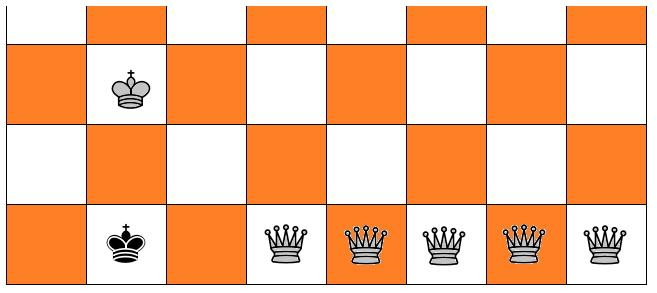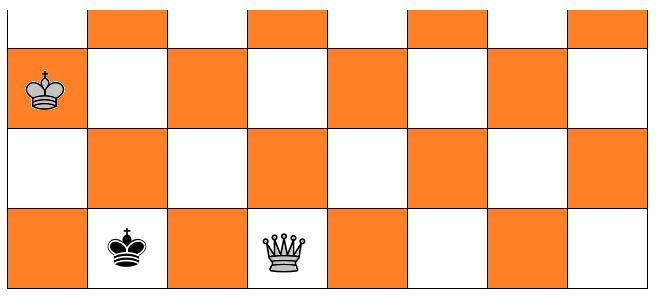# Checkmate Puzzle

The white king is randomly placed in the 3rd row, the black king in the 1st, the white queen either in the 2nd or elsewhere in the 1st. What is the probability that the pieces are in a checkmate position?The white king is randomly placed in the 3rd row, the black king in the 1st, the white queen either in the 2nd or elsewhere in the 1st. What is the probability that the pieces are in a checkmate position?

This is clearly a counting problem in which the probability of an event is determined in the classical manner, as the ratio of the number of successful outcomes to the number of all possible outcomes. The latter is immediate: the white king may be in eight positions in the 3rd row. Similarly, the black king may be in one of eight positions in the first row. The white queen is either in one of the eight positions in the 2nd row or in one of the remaining seven in the first. By the Product Rule, the total number of possibilities is

8×8×(8 + 7) = 960.

The task is to count the number of the successful outcomes, i.e., the number of positions in which the black king is checkmated. There are several cases: two for the black king in a corner, and three for the black king elsewhere.

### The black king in a corner

For each corner, the white pieces may be in sixteen positions (2×16 = 32):For each corner, there is one extra position (+2):### The black king not in a corner

Five positions when the kings are in opposition (+6×5 = 30):Three positions with the white queen in the second row (+6×3 = 18):Five positions where the kings are one vertical position apart and the black king blocked by the queen from moving into the second row. This could happen in both directions (+2×5 = 10):All in all, the number of checkmate positions is 32 + 2 + 30 + 18 + 10 = 92. The probability of this happening is 92/960 = 23/240.

### Reference

1. A. A. Sveshnikov, Problems in Probability Theory, Mathematical Statistics and Theory of Random Functions, Dover, 1968, #2.5